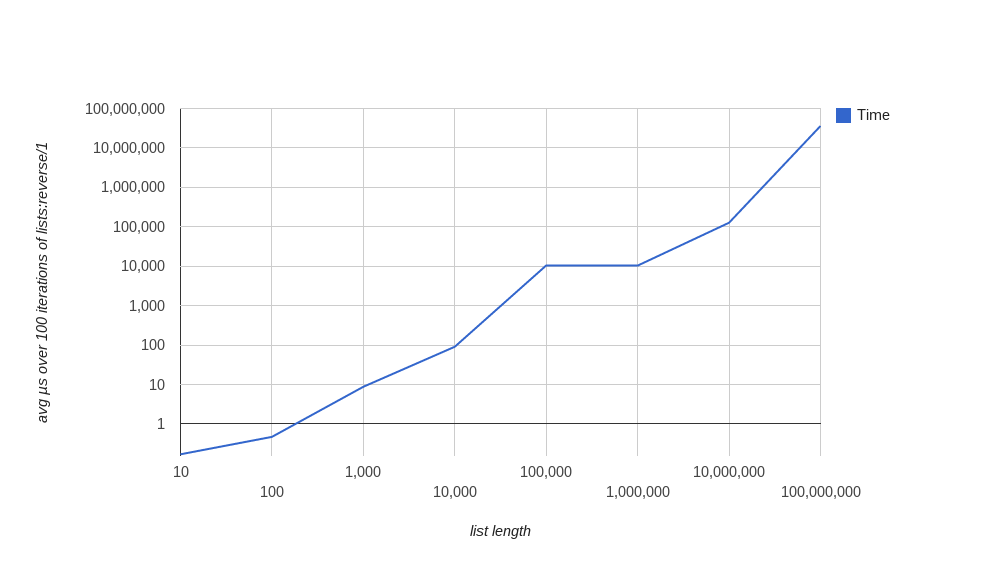## lists:reverse/1 performance in Erlang

| categories:

A common pattern in Erlang is to do some work on a list and accumulate results in "reverse order":

``````do_work(List) ->
do_work(List, []).

do_work([], Acc) ->
Acc;
do_work(Tail, [Result | Acc]).
``````

If you want to preserve the ordering of the input list, you need to reverse the accumulator before return:

``````do_work([], Acc) ->
lists:reverse(Acc);
``````

A question that comes up (in this case on #erlang today) is how well lists:reverse/1 performs: when should we reach for another data structure?

Because this pattern is so common, lists:reverse/2, which lists:reverse/1 is written in terms of, is a BIF: definition in C (note that a pile of other list functions are defined in C in the same file).

This means it should run pretty fast. The Erlang efficiency guide mentions it in the context of tail recursion, but urges us to measure.

I did some simple measurement on an old Xeon E5620 running Linux:

``````-module(reverse_bench).
-export([run/0]).

run() ->
Steps = [10, 100, 1000, 10000, 100000, 1000000, 10000000, 100000000],
Sequences = [{N, lists:seq(1, N)} || N <- Steps],
Timings = [{N, avg_reverse_timing(Seq, 100)} || {N, Seq} <- Sequences],
io:format("~p~n", [Timings]).

avg_reverse_timing(List, N) ->
Timings = repeat_tc(lists, reverse, [List], N, []),
lists:sum(Timings) / length(Timings).

repeat_tc(_, _, _, 0, Acc) ->
Acc;
repeat_tc(M, F, A, N, Acc) ->
{Timing, _} = timer:tc(M, F, A),
repeat_tc(M, F, A, N - 1, [Timing | Acc]).
``````

and graphed it with the Google Charts API:.

Some log scales here, but as you can see, our list needs to be 100,000 elements before we hit 10ms average on this system: assuming we're doing something interesting when we process each list element, lists:reverse/1 is unlikely to dominate our runtime. Besides, since we mostly work with much smaller lists in real programs, this is definitely in the realm of "don't worry".

It's not super-scientific, but the simple answer to when to reach for something other than a list appears to be "not quickly." :o) Good luck, and measure: even naive measurement beats guessing.